Courses

# Design of Power Transmission System - MCQ Test 1

## 20 Questions MCQ Test GATE Mechanical (ME) 2022 Mock Test Series | Design of Power Transmission System - MCQ Test 1

Description
This mock test of Design of Power Transmission System - MCQ Test 1 for Mechanical Engineering helps you for every Mechanical Engineering entrance exam. This contains 20 Multiple Choice Questions for Mechanical Engineering Design of Power Transmission System - MCQ Test 1 (mcq) to study with solutions a complete question bank. The solved questions answers in this Design of Power Transmission System - MCQ Test 1 quiz give you a good mix of easy questions and tough questions. Mechanical Engineering students definitely take this Design of Power Transmission System - MCQ Test 1 exercise for a better result in the exam. You can find other Design of Power Transmission System - MCQ Test 1 extra questions, long questions & short questions for Mechanical Engineering on EduRev as well by searching above.
QUESTION: 1

Solution:
QUESTION: 2

Solution:
QUESTION: 3

### Tooth interference in an external in volute spur gear pair can be reduced by

Solution: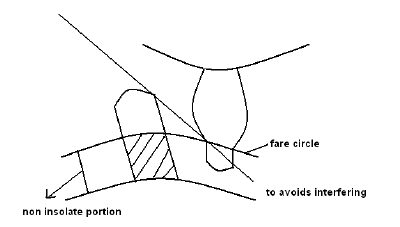There are several ways to avoid interfering:

i. Increase number of gear teeth

ii. Modi fied involu tes

iii. Modif ied adden dum

iv. Increased centre distance

QUESTION: 4

Twenty degree full depth involute profiled 19-tooth pinion and 37-tooth gear are in mesh. If the module is 5 mm, the centre distance between the gear pair will be      [GATE-2006]

Solution: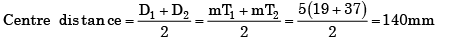QUESTION: 5

A 20° full depth involute spur pinion of 4 mm module and 21 teeth is to transmit 15 kW at 960 rpm. Its face width is 25 mm.

The tangential force transmitted (in N) is

Solution:

P =15 kW
m = 4 mm
Zp = 21
N = 960 rpm
b = 25mm
Dp =mZp = 4 × 21 = 84 mm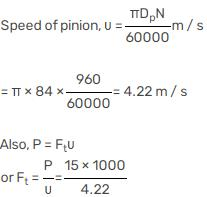≈ 3552 N

QUESTION: 6

A gear set has a opinion with 20 teeth and a gear with 40 teeth. The pinion runs at 0 rev/s and transmits a power of 20 kW. The teeth are on the 20° full –depth system and have module of 5 mm. The length of the line of action is 19 mm.

The center distance for the above gear set in mm is

Solution:
QUESTION: 7

The overall gear ratio in a 2 stage speed reduction gear box (with all spur gears) is 12. The input and output shafts of the gear box are collinear. The countershaft which is parallel to the input and output shafts has a gear (Z2 teeth) and pinion (Z3 = 15 teeth) to mesh with pinion (Z1 = 16 teeth) on the input shaft and gear (Z4 teeth) on the output shaft respectively. It was decided to use a gear ratio of 4 with 3 module in the first stage and 4 module in the second stage.

Que: Z2 and Z4 are

Solution: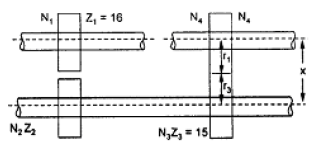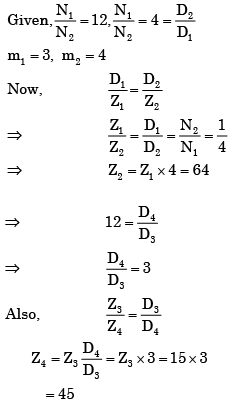QUESTION: 8

The overall gear ratio in a 2 stage speed reduction gear box (with all spur gears) is 12. The input and output shafts of the gear box are collinear. The countershaft which is parallel to the input and output shafts has a gear (Z2 teeth) and pinion (Z3 = 15 teeth) to mesh with pinion (Z1 = 16 teeth) on the input shaft and gear (Zteeth) on the output shaft respectively. It was decided to use a gear ratio of 4 with 3 module in the first stage and 4 module in the second stage.

The centre distance in the second stage is

Solution:
QUESTION: 9

An epicyclic gear train is shown schematically in the adjacent figure.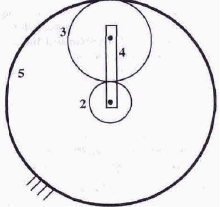The sun gear 2 on the input shaft is a 20 teeth external gear. The planet gear 3 is a 40 teeth external gear. The ring gear 5 is a 100 teeth internal gear. The ring gear 5 is fixed and the gear 2 is rotating at 60 rpm (ccw = counter-clockwise and cw = clockwise). The arm 4 attached to the output shaft will rotate at

Solution:
QUESTION: 10

The sun gear in the figure is driven clockwise at 100 rpm. The ring gear is held stationary.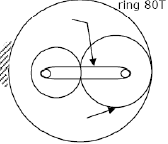For the number of teeth shown on the gears, the arm rotates at

Solution: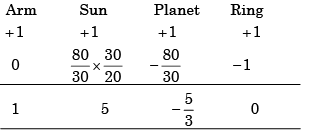For 5 Revolutions Of Sun , Arm rotates by1

∴ for 100 revolutions of Sun,Arm rotates by = 100/5 = 20

QUESTION: 11

For (ω1 = 60 rpm clockwise (cw) when looked from the left, what is the angular velocity of the carrier and its direction so that Gear 4 rotates in counter clockwise (ccw) direction at twice the angular velocity of Gear 1 when looked from the left

Solution: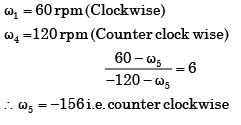QUESTION: 12

Large speed reductions (greater than 20) in one stage of a gear train are possible through

Solution:
QUESTION: 13

To make a worm drive reversible, it is necessary to increase

Solution:
QUESTION: 14

Consider the following statements:

When two gears are meshing, the clearance is given by the

1. Difference between dedendum of one gear and addendum of the mating gear.

2. Difference between total and the working depth of a gear tooth.

3. Distance between the bottom land of one gear and the top land of the mating gear.

4. Difference between the radii of the base circle and the dedendum circle.

Which of these statements are correct?

Solution:
QUESTION: 15

Match List I with List II and select the correct answer using the codes given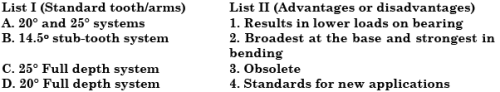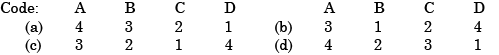Solution:
QUESTION: 16

Match List I with List II and select the correct answer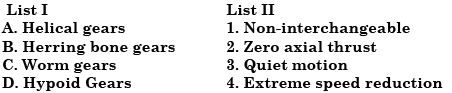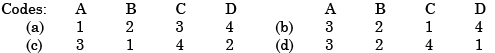Solution:
QUESTION: 17

Match List I (Type of Gear/Gear Train) with List II (Different Usage and Drive) and select the correct answer using the code given below the Lists: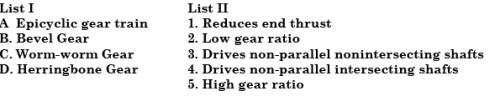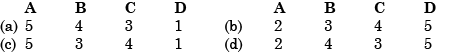Solution:
QUESTION: 18

The gears employed for connecting two non-intersecting and non-parallel, i.e., non-coplanar shafts are

Solution:
QUESTION: 19

Which one of the following pairs is not correctly matched?

Solution:
QUESTION: 20

Gearing contact is which one of the following?

Solution:

When pair of teeth touch at the pitch point ,they have for the instant pure rolling action. At any other position they have the slidingaction.